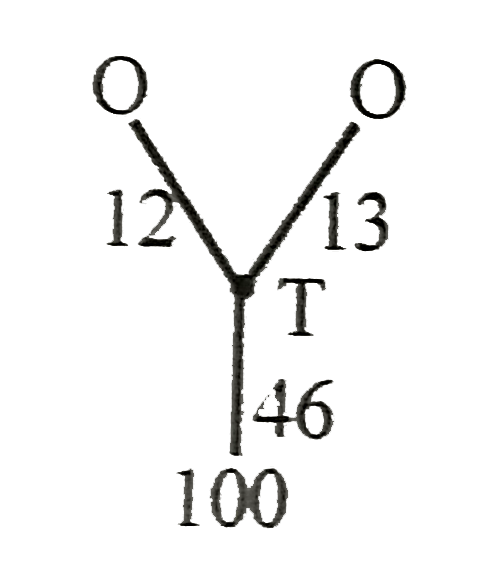# Three rods of Copper, Brass and Steel are welded together to from a Y shaped structure. Area of cross-section of each rod =4cm^2. End of copper rod

25 views
in Physics
closed
Three rods of Copper, Brass and Steel are welded together to from a Y shaped structure. Area of cross-section of each rod =4cm^2. End of copper rod is maintained at 100^@C where as ends of brass and steel are kept at 0^@C. Lengths of the copper, brass and steel rods are 46, 13 and 12 cm respectively. The rods are thermally insulated from surroundings excepts at ends. Thermal conductivities of copper, brass and steel are 0.92, 0.26 and 0.12 CGS units respectively. Rate of heat flow through copper rod is :
A. 2.4cal//s
B. 4.8cal//s
C. 6.0cal//s
D. 1.2cal//s

by (90.1k points)8xx((100-T))/((46)/(0.92xx4))=((T-0)/(13))/(0.26xx4)+((T-0)/(12))/(0.12xx4)
0.08(100-T)=0.08T+0.09T
8=0.11T
T=40
(dH)/(dt)=60xx0.08=4.8erg//s